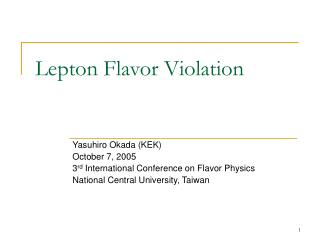DownloadDownload PresentationLepton Flavor Violation

# Lepton Flavor Violation

Télécharger la présentation## Lepton Flavor Violation

- - - - - - - - - - - - - - - - - - - - - - - - - - - E N D - - - - - - - - - - - - - - - - - - - - - - - - - - -
##### Presentation Transcript

1. Lepton Flavor Violation Yasuhiro Okada (KEK) October 7, 2005 3rd International Conference on Flavor Physics National Central University, Taiwan

2. Lepton Flavor Violation in charged lepton processes • Charged lepton LFV is a clear evidence of physics beyond the Standard Model. • Neutrino mixing suggests existence of lepton flavor mixing. Large LFV in charged lepton processes is possible, if there is new physics at the TeV scale. • There are various processes and observable quantities in muon and tau LFV processes. These are keys to choose a correct model among various candidates.

3. Contents of this talk • Phenomenology of muon and tau LFV processes. • Two examples (1) Muon LFV processes in SUSY models including Higgs exchange contributions. (R.Kitano and Y.O) (2) Muon and tau LFV processes in the Left-Right symmetric model. (A.Akeroyd, M.Aoki, and Y.O)

4. Three muon LFV processes m-e conversion search at 0(10^{-18}) will be possible in a future experiment at J-PARC (PRIME)

5. m-> 3e Various observables in mu LFV processes Angular distribution in polarized muon LFV processes m-> eg Example : A= -1 for the SUSY seesaw model Two P-odd and one T-odd asymmetries

6. :dipole :scalar :vector Z dependence of mu-e conversion processes. Z-dependence can distinguish different LFV Interactions. R.Kitano, M.Koike and Y.O. 2002

7. Tau LFV processes Various flavor structures and their CP conjugates

8. Experimental upper bound Y.Miyazaki ESP 2005 Most of processes are bounded by 0(10^-7)

9. Future experimental prospects for tau LFV search Super KEKB LoI e+e- B factory

10. “Polarized” tau decay R.Kitano and Y.O. 2001 Using angular correlation of tau decay products in signal and opposite sides, asymmetries of “polarized” tau decay are obtained. A=-1 case Example: Similarly, two P-odd and one T-odd asymmetries can be defined for tau -> lll decays.

11. m LFV in SUSY models In many models of SUSY, off-diagonal terms of the slepton mass matrixes are induced from Interaction at GUT /seesaw neutrino scales. F.Borzumati, A.Masiero 1986 In many cases, LFV processes are dominated by the m->e g amplitude.

12. m e s s Enhancement due to Higgs exchange contribution at large tan b case Higgs exchange diagram have a ( tan b )^6 contribution. We have shown impact of this contribution in the SUSY seesaw model (LL case). R.Kitano, M. Koike and S. Komine and Y.O. 2003 Higgs-exchange contribution Photon-exchange contribution Dominance of the Higgs exchange affects the Z dependence dipole, scalar, vector

13. Higgs exchange contribution in general case. R.Kitano and Y.O. LL:Flavor mixing in the left-handed slepton sector (ex. SUSY seesaw model) RR: Flavor mixing in the right-handed slepton sector LR, RL: Flavor mixing in the A term RR LL

14. LR RL Higgs exchange effect is evident for the LL case. Situation is more complicated in the RR case due to possible cancellation in both m -> eg and m-e conversion amplitudes. Higgs exchange effects are seen in LR/RL cases.

15. m/t LFV in LR symmetric model A.Akeroyd, M.Aoki, Y.O. (Non-SUSY) left-right symmetric model V.Cirigliano, A.Kurylov, M.J.Ramsey-Musolf, P.Vogel, 2004 O.M.Boyarkin, G.G.Boyarkina, T.I.Bakanova, 2004 L<->R parity Higgs fields, (bi-doublet, two triplets) Low energy (TeV region ) seesaw mechanism for neutrino masses

16. Four lepton interactions are dominant among various LFV processes. In general, We consider following questions: Which is important, m->3e or t ->lll ? What are sizes of various tau decay modes? What is the asymmetry of the tau LFV decay? Heavy particles at the TeV region

17. Relationship between tau and mu decays depends on heavy neutrino Masses. Hierarchical heavy neutrino masses -> large tau decay processes Blue MR3 can be much Heavier than MR1 and MR2. Pink: nearly degenerate Heavy neutrinos

18. Case 2 Case 1 Dominant modes depend on flavor mixings in the heavy neutrino sector.

19. Independent of LFV decay modes including m-> 3e. • Summary • Tau vs. mu : • heavy neutrino mass hierarchy • (2) Various tau modes: • flavor mixing in heavy neutrino sector • (3) t ->3l (or m -> 3e) asymmetry: • ratio of the left and right doubly charged Higgs boson masses.

20. Summary • Experimental Improvement is expected in coming years for m -> eg (MEG) and various tau LFV processes (B factory, LHC). • If there are new particles at the TeV region related to the lepton flavor mixing, the neutrino oscillation can have connection to the charged lepton LFV processes. • There are various observable quantities in muon and tau LFV processes. Different neutrino mass generation mechanism predicts different characteristic signals in LFV processes.